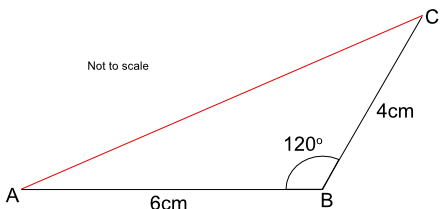Drawing Diagrams from Descriptions

# Drawing Diagrams from Descriptions

GCSE(F),

A ruler is used for measuring distances and drawing straight lines.

A protractor is used for measuring angles. Make sure that the centre of the protractor (the cross-hairs) is on the point of the angle, and that one of the zero lines lies along one of the angle lines.

A pair of compasses are for drawing radii. the radii may be for a circle of a given radius, or may be an arc (when used for creating an intersection point a given distance from a point).

Set squares - triangular shapes from a geometry set - are used on engineering drawings to draw parallel lines. Both set squares will have a 90º angle: one set square will have angles of 45º/45º, and the other 30º/60º.

Dividers - which look like a pair of compasses but with two point and no pencil - are used to measure distances.

## Examples

1. Two lines AB and BC intersect at B with an angle of 120º as shown in the diagram. Line AB is 6cm long, and line BC is 4cm long. By accurately drawing the lines, determine the length of the line AC.Answer: 9.2cm

Draw the line AB 6cm long.

At point B, measure an angle of 120º.

Draw a line from B through the measured angle.

Measure 4cm along this line and mark 4cm.

Measure the line AC.

2. By drawing the diagram, measure the angle ACB.